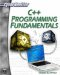# Statements and Expressions

As you have already seen, a statement is simply a single line of code that performs some action. Remember that another word for a statement is an expression. In C++, a single expression does some type of action. That action might be to declare a variable, add two numbers, compare two values, or just about anything at all. It is also important to remember that all statements/expressions end with a semicolon. Another of the most common mistakes that beginners make is leaving off semicolons. Let’s look at a few simple statements.

`int i_acctnum; i_acctnum = 555555; i_acctnum = i_acctnum + 5;`

Each statement performs a different action—but it does perform some action—and it ends with a semicolon. The first statement/expression simply declares a variable. The second statement/expression sets that variable equal to some value. Finally, the last statement/expression performs addition and puts the answer in the variable that was previously declared.

### Operators

You saw, at the end of the last section, the use of the + sign. This is an operator. An operator is simply some symbol that performs some action, usually a mathematical action, such as addition or subtraction. C++ supports a number of important operators that you will need to get familiar with. Let’s begin examining C++ operators, starting with the basic math operators, shown in Table 1.4.

Table 1.4: Operators

Operator

Purpose

Example

+

-

To subtract two numbers

*

To multiply two numbers

/

To divide two numbers

++

This is a special operator that simply ments the value by 1. You will see this used later in this book when loops are discussed.

--

This is also a special operator that simply decrements the value by 1.

=

The single equals sign is an assignment operator. It states “make what’s on the left equal to what’s on the right”

==

The double equals is an equality operator. It asks “is what’s on the left equal to what’s on the right?” This is frequently used in if statements (which you will see in a later chapter!)

!=

Not equal to

if (x !=3)

+=

x += 1;

-=

Subtract then assign

x -=1;

||

This is the logical OR operator.

if(j == 5 || j ==10)

&&

This is the logical AND operator

if(j > 5 && j<10)

>>

Bitwise shift to the right

3<<2;

<<

Bitwise shift to the left

3>>2;

&

Bitwise And

3&2;

|

Bitwise Or

3|2

 Watchout! When using the increment (++) or decrement (—) operator, it is necessary to be careful of where you put it. If you put the operator before the variable, then that operation will take place before any other operations in the expression. Some examples follow. `int answer, num; num = 5;`

Now using the expression,

`answer = num++;`

the value of num will first be put in answer, THEN incremented. In other words, the value of answer will be set to 5, and then the value of num will be incremented. If you want num to be incremented BEFORE you assign the value to answer then you must put the increment operator first, as shown in the following example.

`answer = ++num;`

The operators shown in Table 1.4 are your basic math operators and logical operators. There are several other operators you will also see in C++. These will be introduced in later chapters because they are pertinent to topics that will be covered in those chapters. Most of the operators in Table 1.4 should be familiar to you. The math operators represent simple math operations. All math operators occur with a specific order. The order is pretty much the same as the order of operations defined in mathematics. First is * (multiplication), next / (division), then + (addition), and, finally, – (subtraction). The increment ++ and decrement – operators precedence is determined by what side of the variable they are on. You can alter the order of operations by using parentheses, just as you do in mathematics. An example follows.

`answer = x * 3 + 4;`

First, x and 3 would be multiplied, and then 4 would be added to that product. If, instead, you wanted to add 3 and 4, then multiply by x, you could simply use parentheses to denote that.

`answer = x * (3 +4);`

The order of multiplication-division-addition-subtraction is simply the order defined by mathematics for these operations. Some teachers even use the mnemonic My Dear Aunt Sally to help students to recall the proper order of operations. The important thing to remember is that an operator is simply a symbol that defines some action to be taken. The + symbol defines the action of addition and the \ symbol defines the action of division. Other operators, such as the increment ++ operator, may be new to you, but the fact remains that they are simply symbols that define some action.

An operator is considered to be a unary operator if it takes only one argument. Put another way, if a symbol only acts on one number then it is a unary operator. The increment and decrement operators fall into this category. They only work on a single variable. Binary operators, on the other hand, work on two numbers. Addition and subtraction both work on two numbers. A more technical definition would be that binary operators are operators that take two arguments.C++ Programming Fundamentals (Cyberrookies)
ISBN: 1584502371
EAN: 2147483647
Year: 2005
Pages: 197
Authors: Chuck Easttom